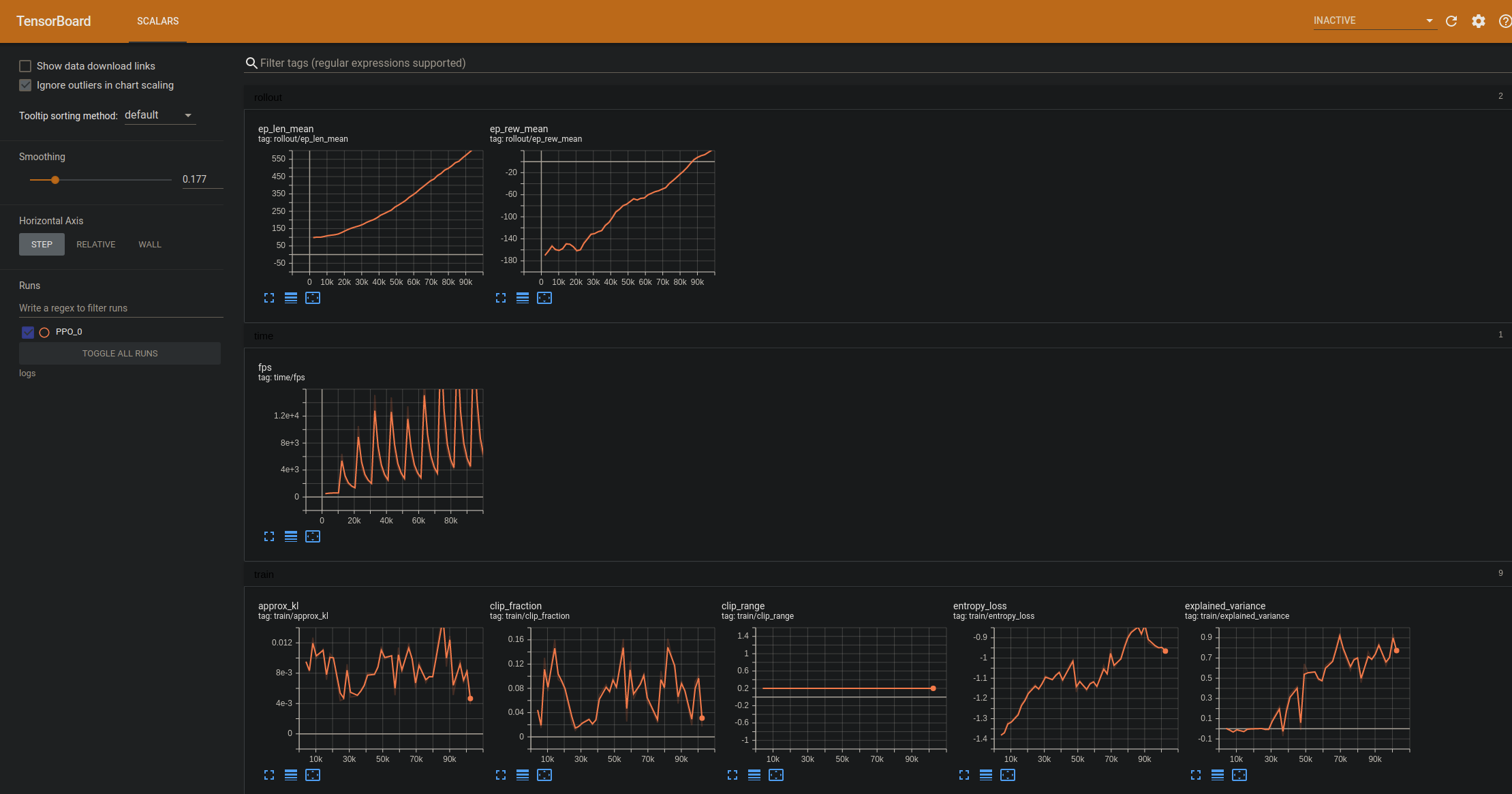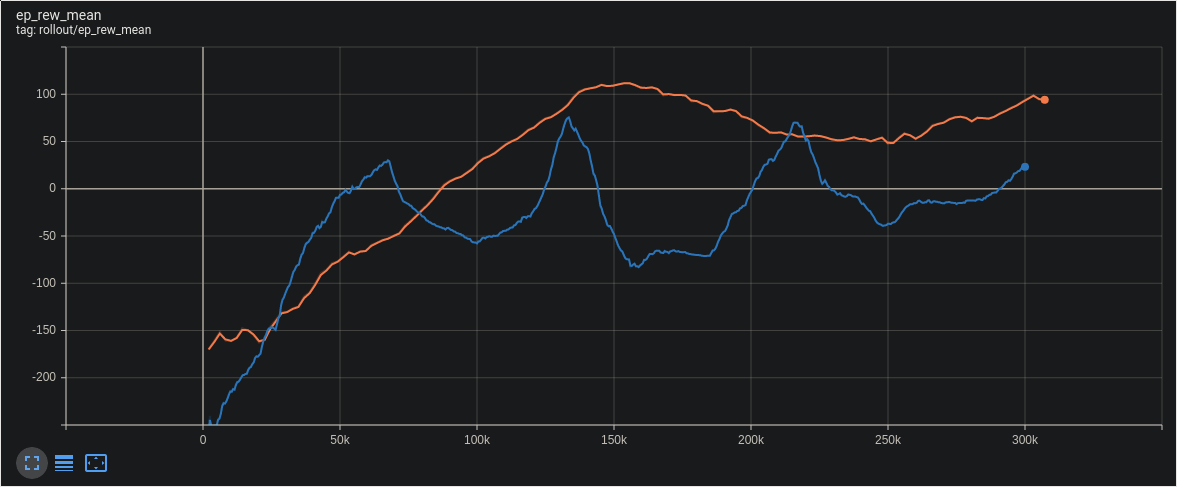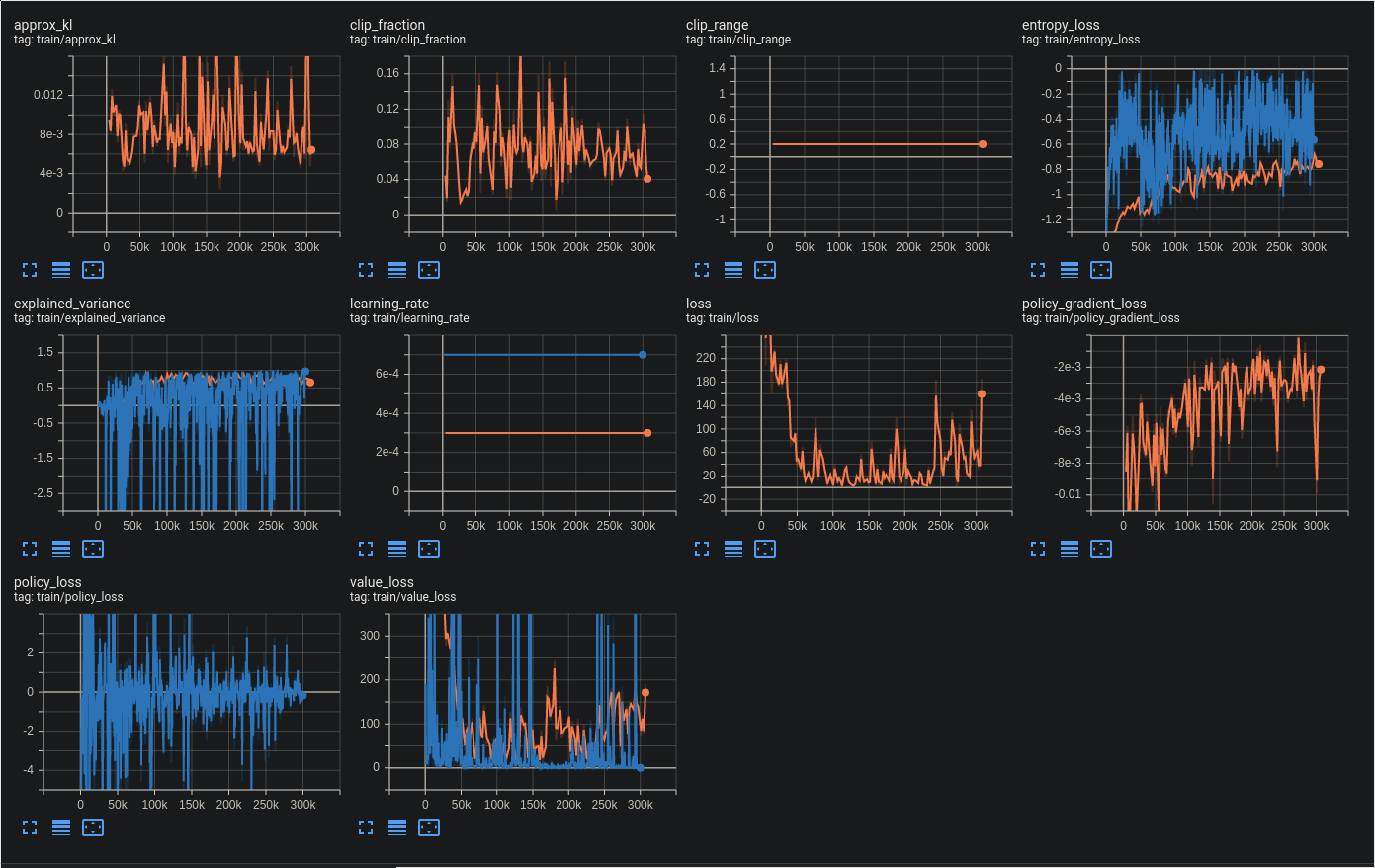## Reinforcement Learning in Python with Stable Baselines 3

#### How to save and load models

Welcome to part 2 of the reinforcement learning with Stable Baselines 3 tutorials. We left off with training a few models in the lunar lander environment. While this was beginning to work, it seemed like maybe even more training would help. How much more? When we trained for 10,000 steps, and decided we wanted to try 100,000 steps, we had to start all over. If we want to try 1,000,000 steps, we'll also need to start over. It makes a lot more sense to save models along the way, and to probably just train until you're happy with the model or want to change it in some way, which will require starting over. With this in mind, how might we save and load models?

Our training code up to this point:

```import gym
from stable_baselines3 import PPO

env = gym.make('LunarLander-v2')
env.reset()

model = PPO('MlpPolicy', env, verbose=1)
model.learn(total_timesteps=100000)```

Let's decrease the timesteps to 10,000 instead, as well as create a models directory:

```import os

models_dir = "models/PPO"

if not os.path.exists(models_dir):
os.makedirs(models_dir)```

Then, we'll encase our training in a while loop:

```TIMESTEPS = 10000
while True:
model.learn(total_timesteps=TIMESTEPS, reset_num_timesteps=False)```

Note the `reset_num_timesteps=False`. This allows us to see the actual total number of timesteps for the model rather than resetting every iteration. We're also setting a constant for however many timesteps we want to do per iteration. Now we can save with `mode.save(PATH)`. I propose we track however many iterations we've made, and then calculate what timestep that model is, saving that as the name:

```TIMESTEPS = 10000
iters = 0
while True:
iters += 1
model.learn(total_timesteps=TIMESTEPS, reset_num_timesteps=False)
model.save(f"{models_dir}/{TIMESTEPS*iters}")```

Full code up to this point:

```import gym
from stable_baselines3 import PPO
import os

models_dir = "models/PPO"

if not os.path.exists(models_dir):
os.makedirs(models_dir)

env = gym.make('LunarLander-v2')
env.reset()

model = PPO('MlpPolicy', env, verbose=1)

TIMESTEPS = 10000
iters = 0
while True:
iters += 1

model.learn(total_timesteps=TIMESTEPS, reset_num_timesteps=False)
model.save(f"{models_dir}/{TIMESTEPS*iters}")```

Let's go ahead and run that for a bit, you should see every ~10,000 timesteps a model will be saved. You can just keep an eye on the `ep_rew_mean`, waiting for something positive, or just go make some coffee or something while you wait for the model to hopefully do something. At least for me, around 110,000 steps, the agent began scoring an average in the positives:

```    ------------------------------------------
| rollout/                |              |
|    ep_len_mean          | 628          |
|    ep_rew_mean          | 4.46         |
| time/                   |              |
|    fps                  | 6744         |
|    iterations           | 4            |
|    time_elapsed         | 16           |
|    total_timesteps      | 110592       |
| train/                  |              |
|    approx_kl            | 0.0025332319 |
|    clip_fraction        | 0.0189       |
|    clip_range           | 0.2          |
|    entropy_loss         | -0.975       |
|    explained_variance   | 0.759        |
|    learning_rate        | 0.0003       |
|    loss                 | 2            |
|    value_loss           | 29.1         |
------------------------------------------
```

By 150,000 steps, the model appears to be on to something:

```    -----------------------------------------
| rollout/                |             |
|    ep_len_mean          | 783         |
|    ep_rew_mean          | 80.6        |
| time/                   |             |
|    fps                  | 9801        |
|    iterations           | 4           |
|    time_elapsed         | 15          |
|    total_timesteps      | 151552      |
| train/                  |             |
|    approx_kl            | 0.013495723 |
|    clip_fraction        | 0.0808      |
|    clip_range           | 0.2         |
|    entropy_loss         | -0.723      |
|    explained_variance   | 0.719       |
|    learning_rate        | 0.0003      |
|    loss                 | 4.06        |
|    value_loss           | 48.3        |
-----------------------------------------
```

We have these models saved, so we might as well let things continue to train as long as the model is improving quickly. While we wait, we can start programming the code required to load and actually run the model, so we can see it visually. In a separate script, the main bit of code for loading a model is:

```env = gym.make('LunarLander-v2')  # continuous: LunarLanderContinuous-v2
env.reset()

model_path = f"{models_dir}/250000.zip"

Then, we can use the same code as we used before to play the model from part one, making our full code:

```import gym
from stable_baselines3 import PPO

models_dir = "models/PPO"

env = gym.make('LunarLander-v2')  # continuous: LunarLanderContinuous-v2
env.reset()

model_path = f"{models_dir}/250000.zip"

episodes = 5

for ep in range(episodes):
obs = env.reset()
done = False
while not done:
action, _states = model.predict(obs)
obs, rewards, done, info = env.step(action)
env.render()
print(rewards)```This appears to me to be "solved" at this point, and it only took a few minutes! It wont always be this easy, but you can probably stop training this model now. On a more realistic problem, you will likely need to go for millions...or billions... of steps, and that's if things work at all. You'll also probably need to tweak things like the reward function. But, for now, let's take the win!

While we can see our model's stats in the console, it can often be hard to really visualize where you are in the training process, and it can be even harder when you're going to be comparing multiple models. The next thing we can do to make this easier is to log model performance with Tensorboard. To do this, we just need to specify a name and location for the Tensorboard logs. First, we'll make sure the log dir exists:

```logdir = "logs"

if not os.path.exists(logdir):
os.makedirs(logdir)```

Next, when specifying the model, we can pass the log directory:

`model = PPO('MlpPolicy', env, verbose=1, tensorboard_log=logdir)`

As we train, we can name the individual log:

`    model.learn(total_timesteps=TIMESTEPS, reset_num_timesteps=False, tb_log_name="PPO")`

Finally, let's limit the number of steps to 300K. Making the full code now:

```import gym
from stable_baselines3 import PPO
import os

models_dir = "models/PPO"
logdir = "logs"

if not os.path.exists(models_dir):
os.makedirs(models_dir)

if not os.path.exists(logdir):
os.makedirs(logdir)

env = gym.make('LunarLander-v2')
env.reset()

model = PPO('MlpPolicy', env, verbose=1, tensorboard_log=logdir)

TIMESTEPS = 10000
iters = 0
for i in range(30):
model.learn(total_timesteps=TIMESTEPS, reset_num_timesteps=False, tb_log_name="PPO")
model.save(f"{models_dir}/{TIMESTEPS*i}")```

Now, while the model trains, we can view the results over time by opening a new terminal and doing: `tensorboard --logdir=logs`Now we can compare to other algorithms to see which one performs better. Let's try A2C again by changing the import, model definition, and log name:

```import gym
from stable_baselines3 import A2C
import os

models_dir = "models/A2C"
logdir = "logs"

if not os.path.exists(models_dir):
os.makedirs(models_dir)

if not os.path.exists(logdir):
os.makedirs(logdir)

env = gym.make('LunarLander-v2')
env.reset()

model = A2C('MlpPolicy', env, verbose=1, tensorboard_log=logdir)

TIMESTEPS = 10000
iters = 0
for i in range(30):
model.learn(total_timesteps=TIMESTEPS, reset_num_timesteps=False, tb_log_name="A2C")
model.save(f"{models_dir}/{TIMESTEPS*i}")
```

Now, it should be much more clear the differences between PPO and A2C. The most obvious difference is A2C seems far more volatile in the reward over time.We can see this volatility elsewhere as well in the other training stats:The next tutorial: